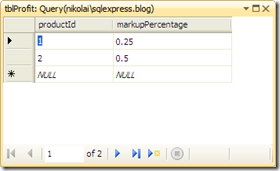I am not a SQL DBA but like a lot of developers I do get to work in relational databases. Also I guess like a lot of developers I find SQL to be the most alien of the languages I work in.

Recently I needed to refactor some code that had an impact on a database, in common with the DRY techniques I wanted to remove duplicated calculations. I’ve simplified this example to make it easier to read.

Data needs to be returned from a SQL Server database to the web site DAL, the code in the DAL used ExecuteScalar to get results back from the stored procedure. We need to calculate the profit for a given product and net price combination as well as being able to calculate the total cost

The profit for each product is held on a table like thisThe calculation for the profit is performed by a procedure like this

```ALTER PROCEDURE dbo.prGetProfit
(
@productId int,
@netPrice real
)
AS
declare @markupPercentage as real, @profit as real

set @markupPercentage = (select markupPercentage
from tblProfit
where productId = @productId)

set @profit = @netPrice * @markupPercentage

select @profit as  profitAC

return @profit```

When run in Visual Studio it looks like this

```Running [dbo].[prGetProfit] ( @productId = 1, @netPrice = 100 ).

profit
25

No rows affected.
(1 row(s) returned)
@RETURN_VALUE = 25
Finished running [dbo].[prGetProfit].
```

All is good, to implement the total cost we could implement the profit logic again and add back on the net price however we want to eliminate the duplicated logic. At first glance this looks easy we could write a procedure like this

```ALTER PROCEDURE dbo.prGetTotalPriceFirstAttempt
(
@productId int,
@netPrice real
)
AS

declare @totalcost as real, @profit as real

exec @profit = prGetProfit
@productId = @productId,
@netPrice = @netPrice

set @totalcost = @netPrice + @profit

select @netPrice + @profit as totalCost```

and run it like this

```Running [dbo].[prGetTotalPriceFirstAttempt]
( @productId = 1, @netPrice = 100 ).

profit
25

No rows affected.
(1 row(s) returned)
totalCost
125

No rows affected.
(1 row(s) returned)
@RETURN_VALUE = 0
Finished running [dbo].[prGetTotalPriceFirstAttempt].
```

However if you look carefully in the output from calling the procedure the first returned value is the profit, then the total cost is returned and its the first value that gets returned into the DAL.

What we need to do is rewrite the EXEC line to call the prGetProfit stored procedure so that the profit value is calculated and returned correctly, without outputting it. We still need direct calls to prGetProfit to work so we cannot change that stored procedure.

It turns out to be a bit more complex that it looks at first however the following procedure

```ALTER PROCEDURE dbo.prGetTotalPrice
(
@productId int,
@netPrice real
)
AS
declare @totalcost as real, @profit as real

declare @Result TABLE (profit real)

insert into @Result exec prGetProfit
@productId = @productId,
@netPrice = @netPrice

select @profit = profit from @result

set @totalcost = @netPrice + @profit

select @totalcost as totalCost```

when called produces the correct result

```Running [dbo].[prGetTotalPrice] ( @productId = 1, @netPrice = 100 ).

totalCost
125

(1 row(s) affected)
(1 row(s) returned)
@RETURN_VALUE = 0
Finished running [dbo].[prGetTotalPrice].
```

Note that only the total price is returned.